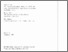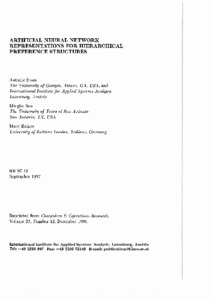# Artificial Neural Network Representations for Hierarchical Preference Structures

Stam, A., Sun, M., & Haines, M. (1996). Artificial Neural Network Representations for Hierarchical Preference Structures. IIASA Research Report (Reprint). IIASA, Laxenburg, Austria: RR-97-012. Reprinted from Computers & Operations Research, 23(12) [December 1996].Preview
Text
RR-97-12.pdf - Published Version

## Abstract

In this paper we introduce two artificial neural network formulations that can be used to assess the preference ratings from the pairwise comparison matrices of the Analytic Hierarchy Process. First, we introduce a modified Hopfield network that can determine the vector of preference ratings associated with a positive reciprocal comparison matrix. The dynamics of this network are mathematically equivalent to the power method, a widely used numerical method for computing the principal eigenvectors of square matrices. However, this Hopfield network representation is incapable of generalizing the preference patterns, and consequently is not suitable for approximating the preference ratings if the pairwise comparison judgments are imprecise. Second, we present a feed-forward neural network formulation that does have the ability to accurately approximate the preference ratings. We use a simulation experiment to verify the robustness of the feed-forward neural network formulation with respect to imprecise pairwise judgments. From the results of these experiment, we conclude that feed-forward neural network formulation appears to be a powerful tool for analyzing discrete alternative multicriteria decision problems with imprecise or fuzzy ratio-scale preference judgments.

Item Type: Monograph (IIASA Research Report (Reprint)) Institute Scholars (INS) Reprinted from Computers & Operations Research; 23(12) [December 1996] IIASA Import 15 Jan 2016 02:07 27 Aug 2021 17:15 http://pure.iiasa.ac.at/4847View Item